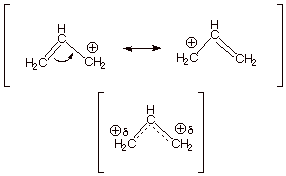# What determines carbocation stability?

Jan 7, 2017

The three factors that determine carbocation stability are adjacent (1) multiple bonds; (2) lone pairs; and (3) carbon atoms.

An adjacent π bond allows the positive charge to be delocalized by resonance.

Thus, $\text{H"_2"C=CHCH"_2^"+}$ is more stable than $\text{CH"_3"CH"_2"CH"_2^"+}$.Resonance delocalization of the charge through a larger π cloud makes the cation more stable.A lone pair on an adjacent atom stabilizes a carbocation.

Thus, $\text{CH"_3"OCH"_2^"+}$ is more stable than $\text{CH"_3"CH"_2"CH"_2^"+}$ because of resonance.

The cation is more stable because the charge is spread over two atoms.

${\text{CH"_3"O"stackrel(+)("C")"H"_2 ⟷ "CH"_3stackrel(+)("O")"=CH}}_{2}$

The stability of carbocations increases as we add more carbon atoms to the cationic carbon.

For example, $\text{CH"_3"CH"_2^"+}$ is more stable than $\text{CH"_3^"+}$

The stabilization is explained by a type of resonance called hyperconjugation.

${\text{H-CH"_2"-"stackrel("+")("C")"H"_2 ⟷ stackrel("+")("H") " CH"_2"=CH}}_{2}$

The ${\text{sp}}^{3}$ orbitals of the adjacent $\text{C-H}$ bonds overlap with the vacant $\text{p}$ orbital on the carbocation.The delocalization of charge stabilizes the carbocation.

Thus, the more alkyl groups there are surrounding the cationic carbon, the more stable it becomes.

That gives us the order of stability: 3° > 2° >1° > 0°